Diodes evaluation for crystal sets, a simple practical approach
by SV3ORA

Recently, I had the chance to own a bunch of germanium diodes. This was the kick-start to begin an experiment for evaluating sensitivity of different types of germanium point contact diodes, for use in the crystal sets.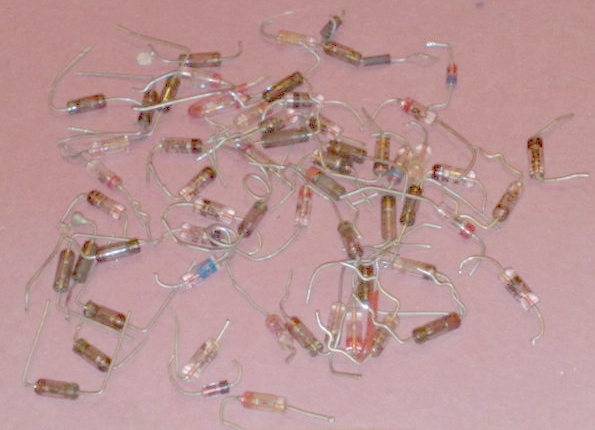The experiment was simple. I connected a very sensitive current meter, shown below, to the germanium test diode and to the ground. On the other end, the diode was connected to a few turns of wire, forming an air coil of about 1cm in diameter. The other end of the coil was connected to the ground.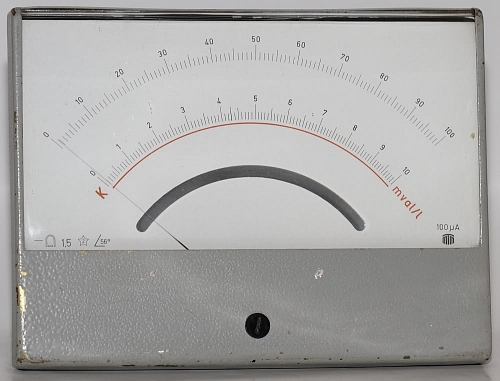The test transmitter was a 446MHz PMR placed about 30-40cm away from the coil. For the experiment, I was standing exactly in the same place each time I turned on the transmitter, as even the slightest movement affects the reading on the meter.

From the 11 types of germanium detector diodes I tested, the winners were:

2. AA119 and OA47, reading current >100uA, just a little bit lower than the OA90.

To give you an indication of the sensitivity, with the same setup, a 1N34A reads only about 20uA.

I have also tested two of my best unmarked diodes and they read the same as AA119. These unknown diodes were tested before on a real crystal set and gave the best sensitivity, thus I assume my readings are quite ok, despite the much higher frequency used. Although, I did not test the diodes for different input power levels.

References: Information from different websites about diodes used as detectors

Website 1

Diodes comparison

All of the diode detectors described above have at least one diode forward voltage-drop in the path to the measuring device. The diode forward voltage drop, Vf, varies approximately in proportion to the logarithm of the current, If, passing through the diode, and also depends on the temperature of the diode junction. For many types of diode, this behavior is described to a good approximation by a modified form of the Ebers-Moll equation:

 Vf = m ( kT/q ) ln[( If / Is ) +1 ]

where k is Boltzmann's constant (1.380662´10Joules/Kelvin) , q is the charge of an electron (1.6021892´10Coulomb), and T is the temperature in Kelvin, i.e., the absolute temperature (°C+273.16). The factor kT/q is sometimes given the symbol VT, and has a value of 25.3mV at 20°C. "m" is a dimensionless correction factor between 1 and 2. "ln" is the natural logarithm (Loge). Is is the junction reverse saturation leakage current.
For small-signal rectifier voltmeters, diodes should be chosen for low forward voltage drop and low junction capacitance. Most sources of information now maintain that the best diodes in this respect are silicon Schottky-barrier (i.e., silicon-metal junction) diodes, such as the 1N5711 (Agilent 5082-2800); and so to investigate this matter, the forward voltage drop versus current characteristics of a variety of small signal diodes were measured. The results are shown in the graph below: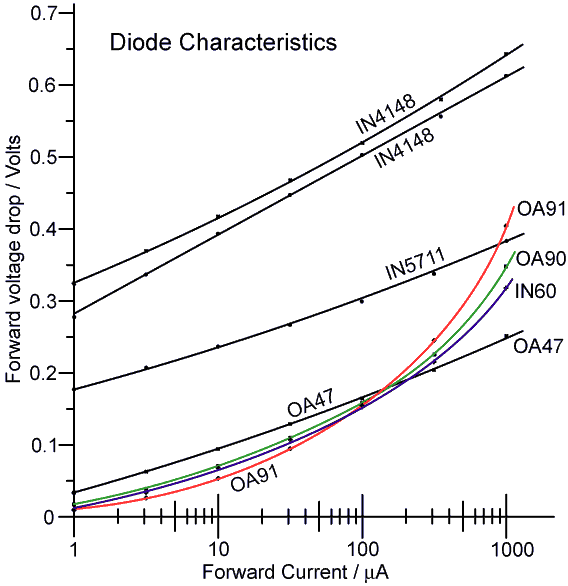The 1N4148 is a silicon P-N junction diode. The 1N5711 is a silicon Schottky diode. The OA47 is an archaic germanium gold-bonded diode, and the rest are germanium point-contact diodes. Two 1N4148 diodes from different manufacturers were measured merely to illustrate the point that silicon P-N diodes have the highest forward voltage drop and are therefore a poor choice for low voltage detectors. The 1N5711 curve is the average of results from four diodes, all from the same batch, which had practically identical characteristics. The OA47 curve is the average for four diodes from two manufacturers, all having similar characteristics. The OA90, OA91, and 1N60 curves are from single examples, and are therefore not necessarily representative of the type. All data were recorded at an ambient temperature of 21°C. The data indicate that the IN4148, the 1N5711, and the OA47, all obey a logarithmic V/I relationship rather well, whereas the germanium point-contact characteristics show considerable curvature due to high internal resistance. With regard to the forward drop however, the germanium diodes are all superior to the IN5711 in the 1-100mA range, and the preference for the latter may merely reflect the fact that many semiconductor manufacturers can no longer fabricate germanium. Silicon Schottky diodes, such as the 1N5711 and 1N6263, being essentially UHF devices, have better high-frequency performance than germanium diodes, but germanium diodes work well at VHF and are therefore perfectly adequate for HF applications. Among the germanium diodes, there is little difference between the gold-bonded and standard varieties in the 1 to 100mA range, but the OA47 is the best choice for currents up to 1mA. We should observe however, that detector diodes only conduct on the peaks of the applied waveform, and so the instantaneous current is much higher than the average current, the difference being about an order of magnitude. Therefore, in selecting diodes for average currents in the region of 1 - 100mA, we should consider the steady-state voltage drop in the region 10mA - 1mA; in which case the germanium gold-bonded diode offers the lowest forward-drop without contest. Note however, that one of the consequences of the Ebers-Moll equation is that low forward voltage-drop is associated with high reverse leakage current. If reverse leakage is an issue, then silicon Schottky diodes are to be preferred. To put this issue in perspective however, the reverse leakage current of an OA47 diode was measured as follows:

 Vr /Volts 1 2 3 5 10 15 20 Ir /mA 1.1 1.3 1.4 2.1 4.1 6.3 8.8

 The leakage current is approximately linear in the 5-20V range and may be modelled by assuming a resistor of about 2MW in parallel with the diode. Such a defect has little effect on the operation of a detector loaded with a 10-100KW resistor. Some final points in favour of the silicon Schottky diodes however, are that germanium diodes show a wider spread of characteristics, and that the OA47 is obsolescent. Hence the Schottky diodes are definitely preferable in applications requiring diode matching, precise calibration, or availability through normal commercial channels. The 1N5711 in particular also, has a very high reverse breakdown voltage for a device of its class, its Vr max of 70V making it suitable for half-wave detectors of up to 24.7V DC ouput. An OA47 half-wave detector has a maximum DC output of 10.6V if Vr max is not to be exceeded.

 Detector diode data:
 Type Description Vr max / V If max / mA If av / mA Typ Vf @ If Typ Ir @ Vr / V / mA / mA / V 1N5711 Si Schottky (2.0pF) 70 - - 0.41* 1 0.2 50 1N5712 Si Schottky (1.2pF) 20 - - 0.55* 1 0.15 16 AA119 Ge point contact 45 100 35 2.6 30 170 45 AAY30 Ge Au-bonded. High speed 30 400 - 0.88 150 8.0 30 AAY32 Ge Au-bonded. High speed 30 150 - 0.60* 30 11 30 AAY33 Ge Au-bonded. High speed 12 240 - 0.5* 30 15 12 AAZ15 Ge Au-bonded. High voltage 100 250 - 0.8 250 16 100 AAZ17 Ge Au-bonded. Gen.purpose 75 250 - 0.8 250 16 75 BAT81 Si Schottky (1.6pF) 40 30 - 0.33 0.41 1.0 0.1 1 15 0.2 30 BAT82 50 BAT83 60 OA47 Ge Au-bonded. Gen.purpose 30 150 - 0.54 30 10 30 OA90 Ge point contact "diode"?** 30 45 10 2.0 30 300 30 OA91 Ge point contact 115 150 50 2.1 30 75 100 OA95 Ge point contact 115 150 50 1.85 30 80 100
 * max. ** a very non-linear resistor pretending to be a diode - best avoided!

For the Omega receiver I have decided to go for the OA47 diode, based on it's obvious advantages over the other diodes. The second generation of the receiver may use a FET detector, but I first have to make a better research on this type of detector. So, for the time being, and to satisfy more classical set readers I will end up to this type of diode.

 Back Diodes: One further rectifying device which we should mention in passing is the backward diode or 'back-diode'. This is a special type of tunnel diode (Esaki diode), which has an extremely low forward voltage drop: in the region of 90mV for 10mA forward current. Unfortunately however, there is no get-out-of-jail-free card as far as the Ebers-Moll equation is concerned: the back diode has an extremely low reverse breakdown voltage, and a leakage of around 1mA for a mere 500mV of reverse bias. The back diode can therefore only be used in extremely low-impedance low-voltage circuits, which comprehensively defeats any advantage it might have had for the construction of linear detectors.

Below I would like to mention two useful things about diodes. The detected voltage as function of the input voltage and the detector diode input impedance

 Detected voltage as function of the input voltage If we rectify a RF signal with a diode we can distinguish two situations. Situation 1: Rectifying in the linear region If the input voltage is high enough (well above the voltage drop of the diode at 1 mA), the output voltage of the diode will be about proportional to the input voltage. So double input voltage, gives about double output voltage. The output voltage is almost equal to the peak value of the input voltage. The power losses in the diode are in this region very low compared to the rectified power. Situation 2: Recifying in the square law region If the input voltage is low, lower then the voltage drop of the diode (at 1 mA) then the situation is completely different. The input of the diode behaves for the RF signal like a resistor with value RD. The output of the diode behaves like a DC voltage source in series with a resistor, the value of this resistor is also equal to RD. The value of the DC voltage source is square law related to the amplitude of the RF input signal. So double input voltage, gives 4 times as much detected DC voltage at the output In the square law region the output voltage of the diode will be much lower then the input voltage, the diode gives much power loss between input and output. The lower the input voltage, the higher the losses. The higher the input voltage, the lower the diode losses. When further increasing the diode input voltage, we gradually come into the linear detection region. When receiving weak stations, detection takes place in the square law region. Between the linear and square law region, there is a region not linear, and not square law but somewhere in between.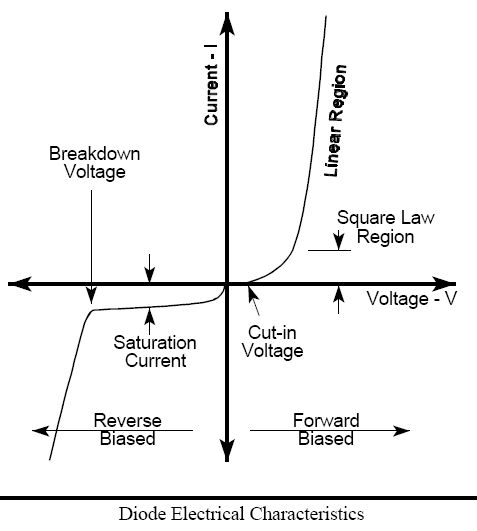Detector diode input impedance A point to which we have so-far only alluded, is that the load presented by the detector has a significant effect on the operation of some types of bridge. We must therefore always keep the detector input impedance in mind when investigating bridge behaviour, and in order to do so we need to have an idea of its value. This is a peculiar problem in the case of a diode detector, because the diode does not obey Ohm's law; and so the input resistance varies during the course of each cycle of the applied voltage. For the most part however, it is sufficient to have an average picture of the detector input impedance, and this can be obtained by considering the power delivered to the detector load resistor RD.
 In the first instance, we can consider the power delivered to RD in the limit that the peak value of the applied voltage Vdet is very large in comparison to the diode forward voltage drop Vf. In this case, the detector output voltage is: Vmeas = Vdet Ö2   and the power delivered to the load resistor is: Pdet = Vmeas˛ / RD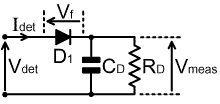Now, all of this power must come from the bridge output. Therefore, if we define the detector input resistance as Rdet, we have:
Pdet = Vdet˛ / Rdet = Vmeas˛ / RD
and using equation (Vmeas = Vdet Ö2) gives:
Vdet˛ / Rdet = (Vdet Ö2)˛ / RD
i.e.,
1 / Rdet = 2 / RD
i.e.,
 Rdet = RD / 2

Thus the limiting input resistance of a simple detector is equal to half the detector load resistance. We can understand this point by thinking of the detector as a kind of voltage transformer. The RMS average of a DC signal is the same as its ordinary average, and so in the process of converting from AC to DC, the RMS level has been transformed-up by a factor of Ö2. Thus the detector is a 1:Ö2 voltage transformer, and hence a 1:(Ö2)˛, i.e., 1:2 impedance transformer.
The limiting input resistance is a reasonable measure of the input impedance when the detector is driven hard, for best linearity. At lower drive levels, the input resistance will be higher, becoming 'infinite' when the peak value of Vdet falls below the forward threshold voltage of the diode (it cannot actually become infinite because Vf also falls as the diode current falls).
The limiting 1:2 impedance transformation rule applies to all non-voltage-multiplying detectors. In the case of a bridge rectifier, the limit is harder to approach because the detector places two diode forward voltage drops in series with the output. In the case of the bi-phase full-wave rectifier (assuming a 1:1:1 transformer ratio) the limit is slightly easier to approach, because the average diode current is shared between two diodes.
Note that the input impedance of a detector will be slightly capacitive at high frequencies. This is due to the junction capacitance of the diode. For the 1N5711 for example, this capacitance is about 2pF.

In the case of a voltage-doubler detector, the limiting output voltage is:
Vmeas = Vdet 2Ö2
Hence:
Pdet = Vdet˛ / Rdet = (Vdet 2Ö2)˛ / RD
i.e.,

 Rdet = RD / 8

Thus the voltage-doubler is a limiting 1:8 impedance transformer. Consequently, it has a much lower input resistance in comparison to a non-multiplying detector for a given value of detector load. The difference is such that advantage of increased output voltage can easily be lost as a result of loading of the driving circuit.
The voltage doubler also has about double the input capacitance of a half-wave rectifier using a given type of diode.

Website 2

### Square Law Diode Detectors in 50 ohm Systems

Most agree that a simple diode peak-detector gives a reasonably accurate method of determining RF power delivered to a load, for powers above about 100mW. Measured DC voltage is simply equal to the peak voltage of the RF waveform: power in watts may be determined by calculating Vpeak2/100....(for 50-ohm systems).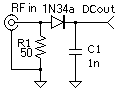For smaller signals, the diode forward voltage (0.6v for silicon, 0.3v for germanium or schottky) causes error: output DC voltage is lower than peak RF voltage. And any diode no longer has a well-defined delineation between conduction and non-conduction for small signals: its rectifying properties become inefficient. Signal levels between a few volts down to a few tens of millivolts require some significant non-linear corrections to be factored in. Detectors such as thermocouples or thermistors (bolometers) can do a better job in this region. We tend to dismiss diodes as error-prone for small signal measurements.
But for really small signals (below about -20dBm) diodes once again can give predictable and useful amplitude measurements. It is useful to divide diode peak-detector operation into three regions: linear (above 20dBm), transition (-20dBm to 20dBm) and square-law (below -20dBm).
What is square law? It simply means that the DC component of diode output is proportional to the square of the AC input voltage. So if you reduce RF input voltage by half, you'll get one quarter as much DC output. Or if you apply ten times as much RF input, you get 100 times as much DC output as you did before. An increase of 3dB results in twice as much output voltage. Square law means that output DC voltage is proportional to RF power delivered to the 50 ohm input terminating resistor. So you could have a linear scale of power (in milliwatts or microwatts or nanowatts) on the scale of the output meter.
There is a downside: square law greatly limits input dynamic range. At -60dBm (223.6uV rms in a 50-ohm system) an efficient detector diode might yield only one microvolt of DC output: not easy to measure.
Note that output is proportional to input2. This proportionality constant depends strongly on how "curved" is the diode's I vs. V curve. Schottky and germanium beat out silicon in this case. Temperature affects the I vs. V curve too, along with the proportionality constant. Much of the utility of our square-law detector will be in relative measurements: if absolute amplitude isn't required, we can ignore the proportionality constant, and forego absolute amplitude calibration. The detector is still very useful. If you want to measure absolute RF input power, you'd have to know the diode's temperature, and do some non-linear corrections of the DC output. This would most likely involve a microcomputer.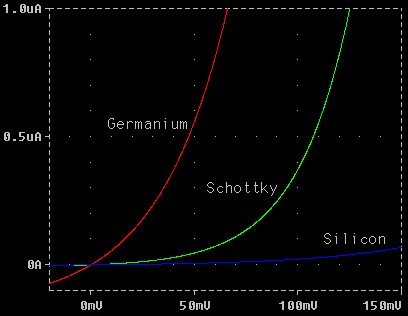What diode to use? All diodes will follow the square-law rule for small signals. We should choose one that is fast, so 1N4000-series power diodes are out. Any diode with a lot of capacitance is out. Diodes with a sharp I vs. V curve will give more output, so silicon diodes are less desirable. Schottky diodes are great, but they require DC biasing to get into the really curved part of the I vs.V curve. With zero bias, a germanium diode has very decent qualities (apart from nasty temperature effects). When Hewlett Packard makes square-law diodes using exotic semiconductors like gallium arsenide with molecular-beam-epitaxy machines, the result looks remarkably similar to a simple point-contact germanium diode like a 1N34A. Only repeatability and stability are much better - they need to measure absolute voltage.
At zero-bias the dynamic resistance of a 1N34A is about 50Kohms (at room temperature). This is the slope of the V vs. I curve at zero volts.  For small signals encountered in square-law, the diode primarily looks like a (50K) resistor which is a bit non-linear, even though all the output depends on the non-linear bit. This means that the diode, and all the circuitry that follows, will load down the 50-ohm input resistance very little. Indeed, we can use two diodes, one for positive swings and the other for negative swings, to double the detected output voltage, and still not affect the 50-ohm termination.
How to measure such small DC output voltage?    Square-law detection is only valid for input signals below about -20dBm. At -20dBm, input voltage across the 50-ohm resistor will only be 63.24 millivolts, peak-to-peak. DC detector output voltage will be even less, and smaller input signals yield ever-smaller output (square-law at work). So the range of detectable input signals depends greatly on how carefully we amplify these small DC voltages. We particularly want to avoid DC offsets: these result in an output where none should exist. For example, in a test setup, a sensitive DC meter capable of displaying in 0.1uV increments showed a DC offset of about -23uV from each diode detector. The source was likely a thermocouple within the RF generator.
In any case, a "zero" control will be required. With no input signal, the zero control will be adjusted to give a zero output voltage. This zeroing process compensates for any offsets, be they from op-amps, or thermocouples. Since most offsets are temperature sensitive,  a change in temperature will likely require re-zeroing. Many modern DC meters will indicate full-scale voltage of about 200mV. With such a meter, we still need to amplify the detector's DC output by a factor of 100 to 10000 times.
What's the RF bandwidth of a diode detector?    Hewlett Packard's diode detectors go up to 18GHz. Our simple germanium diodes are not as good, but the upper bandwidth limit  (-3dB  point) is still a respectable 500MHz. A biased schottky diode such as 1N5711 should do better.

Back to main site